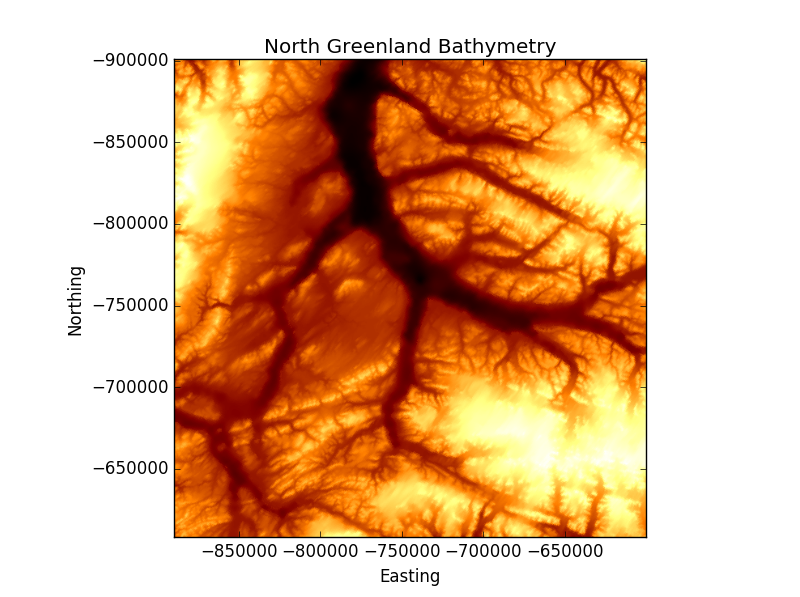# Plotting matrices

There are a number of methods available for plotting grids of data in matplotlib. Start off by saving this file somewhere on your machine.

Let’s start by opening an image - we have to use the `scipy.misc` package for this:

``````from scipy import misc
``````

We have now created a numpy array version of the image (you can check this by typing `type(image)`).

To plot this, we need to use matplotlib.pylot’s `imshow()` function. Now type the following:

``````import matplotlib.pyplot as plt
plt.imshow(image)
plt.show()
``````

This image is RGB - i.e. the array consists of 3 bands where each band holds values between 0-255 (see [here(https://en.wikipedia.org/wiki/RGB_color_model) for more info) making up the red, green and blue components of the image. Remember that you can check the shape of the image array using:

``````image.shape
``````

As the image has RGB information, we can;t override the colour scheme. To do this, we must not present RGB info and we can do this by accessing just one band of the image by slicing the array:

``````image=image[:,:,0]
``````

Now, let’s change the colour scheme - we can do this by setting the `cmap` option - the potential colour schemes can be found here:

``````plt.imshow(image, cmap='cool')
plt.show()
``````

As with the scatter plot (see here), you can also access the following to edit the axis and titles:

``````plt.imshow(image, cmap='cool')
plt.title("Flower ")
plt.xlabel("x dimension")
plt.ylabel("y dimension")
``````

To save the image, you can use the following code to which you pass in the output file which we will associate with a variable call `ofile` (you can call this what you like though):

``````ofile='flower_cool.jpg'
plt.savefig(ofile)
``````

When saving figures, you can also alter a variety of parameters including the dots per inch (dpi) (remember to recreate your figure instance before doing this otherwise the image you save will be empty) e.g.:

``````ofile='flower_cool_300dpi.jpg'
plt.savefig(ofile, dpi=300)
``````

The `plt.savefig()` has a variety of options such as the ability to set transparency so have a look at the documentation to ensure you take full advantage of it.

# Plotting a DEM

Now let’s plot a digital elevation model. Save this file on your machine.

Read it in as an array:

``````dem=misc.imread("/path/to/file/dem.tif")
``````

Create a plot object and apply a sequential colormap (to show the transition from low to high elevations - see here for more info):

``````plt.imshow(dem, cmap='afmhot')
plt.title('North Greenland Bathymetry')
plt.xlabel('Easting')
plt.ylabel('Northing')
plt.show()
``````

The coordinates of the DEM represented by the DEM are:

``````top left (x min):      -889595
top right (y max):     -900955
bottom right (x max):  -600459
bottom left (y min):   -607972
``````

We can use this information to set the tick labels of the image by making use of the `extent` option in `plt.imshow()` e.g.:

``````x_min=-889595
y_max=-900955
x_max=-600459
y_min=-607972
extent_dims=[x_min, x_max, y_min, y_max]
plt.imshow(dem, cmap='afmhot', extent=extent_dims)
``````

This image represents elevation, so let’s make a colorbar to provide useful information to those viewing the image:

``````cbar=plt.colorbar()
``````

Then just show the plot:

``````plt.show()
``````

You should end up with something like this:Have a look at the other options available with `plt.imshow()` and see what else you can do with this figure.